# Exponential Smoothing

Contents[Hide]

The Exponential Smoothing functions apply an exponentially-decreasing weight to historical data in order to forecast future values based on emerging trends.

This function is typically used with a datetime axis but it will also work with a numeric hierarchy or measure for alignment.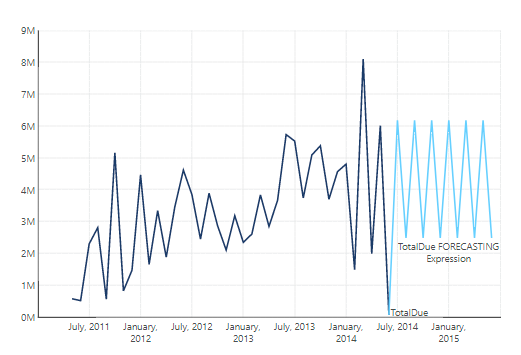Exponential Smoothing

Note
For background information, refer to the OTexts article about exponential smoothing taxonomy and the Nelder-Mead method for optimization fitting.

## 1. Syntax

You can use two forms of exponential smoothing in Dundas BI, the EXPSMOOTH function and the simplified FORECASTING function.

### 1.1. Forecasting

```FORECASTING(d0,d1,s0,s1)
```

Upper Band Error:

```FORECASTING_UPPER(d0,d1,s0,s1)
```

Lower Band Error:

```FORECASTING_LOWER(d0,d1,s0,s1)
```

The same syntax can also be used to return the axis with FORECASTINGAXIS and the season length with FORECASTINGSEASONLENGTH.

### 1.2. Expsmooth

```EXPSMOOTH(d0,d1,s0,s1,s2,s3,s4,s5,s6,s7,s8,s9,s10)
```

Upper Band Error:

`EXPSMOOTH_UPPER(d0,d1,s0,s1,s2,s3,s4,s5,s6,s7,s8,s9,s10)`

Lower Band Error:

`EXPSMOOTH_LOWER(d0,d1,s0,s1,s2,s3,s4,s5,s6,s7,s8,s9,s10)`

The same syntax can be used to return the alpha (EXPSMOOTHALPHA), axis (EXPSMOOTHAXIS), beta (EXPSMOOTHBETA), gamma (EXPSMOOTHGAMMA), mean squared error (EXPSMOOTHMSE), phi (EXPSMOOTHPHI), season length (EXPSMOOTHINGSEASONLENGTH), season type (EXPSMOOTHSEASONTYPE), and trend type (EXPSMOOTHTRENDTYPE).

## 2. Input

The Exponential Smoothing functions require the following input series:

• d0 - Trend hierarchy - The hierarchy over which to perform the forecast (e.g. datetime axis).
• d1 - Base values - The set of historical data values to be used in the formula.

## 3. Parameters

• s0 – Forecast Length – The number of periods to forecast after the end of historical data. A value of -1 indicates the default value, which is 1/3 of the length of the historical data.
• s1 – Season Length – The length of the season, in periods, over which to find seasonal patterns. A value of -1 indicates to use the default, which is automatically selected by Dundas BI.
• s2 – Confidence Level – The probability that the future data will fall within the upper and lower band errors. The default value is 0.95.
• s3 – Show Whole Result – Indicates whether to show only the forecast or the results across all periods. The default is 0 (false).
• s4 – Optimizer Passes – The number of data passes the optimizer should make to find optimal the forecasting values alpha, beta, gamma, and phi. The default value is 10.
• s5 – Trend Smoothing Type – The trend smoothing method to be used. The following options are available:
• -1 – Choose automatically (default)
• 0 – No trend smoothing
• 1 – Additive
• 2 – Additive Damped
• 3 – Multiplicative
• 4 – Multiplicative Damped
• s6 – Season Smoothing Type – The season smoothing method to be used. The following options are available:
• -1 – Choose automatically (default)
• 0 – No trend smoothing
• 1 – Additive
• 2 – Multiplicative
• s7 – Alpha – The level smoothing factor. The default value is optimized (0.25 with optimization disabled). Higher values emphasize more recent data, lower values increase the impact of historical data.
• s8 – Beta – The trend smoothing factor. The default value is optimized (0.25 with optimization disabled). Higher values emphasize more recent trend, lower values increase the impact of broader historical trends.
• s9 – Gamma – The seasonal smoothing factor. The default value is optimized (0.25 with optimization disabled). Higher values emphasize more recent seasonal patterns, lower values increase the impact of historical seasonal patterns.
• s10 – Phi – The trend damping factor. The default value is optimized (0.25 with optimization disabled). Lower values decrease the severity of the forecasted trend when using Additive Damped or Multiplicative Damped trend smoothing types.

## 4. Output

The Exponential Smoothing functions generate the following outputs:

• Exponential Smoothing - The Exponential Smoothing result set.
• Upper Band Error - The upper error boundary based on standard deviation and the forecasting error.
• Lower Band Error - The lower error boundary based on standard deviation and the forecasting error.
• Alpha – The smoothing factor.
• Axis – The forecasting axis.
• Beta – The trend smoothing factor.
• Gamma – The seasonal smoothing factor.
• Mean squared error – The average deviation from historical data.
• Phi – The trend damping factor.
• Season Length – The season length.
• Season type – The season smoothing method used.
• Trend type – The trend smoothing method used.

## 5. Examples

For the following examples of using exponential smoothing, first create a Point chart.

Add a measure (TotalDue), a time hierarchy (OrderDate), and a slicer (OnlineOrderFlag).

Edit the OrderDate hierarchy and change its Level to Month.

Filter the slicer to show only True values.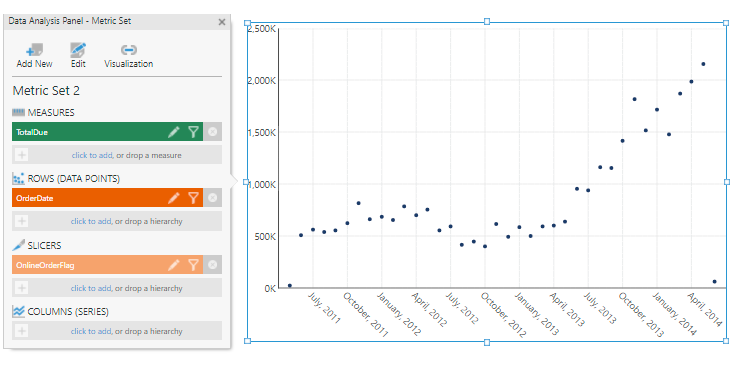Set up the point chart

### 5.1. Single exponential smoothing

For some background information on single exponential smoothing, view this article from the National Institute of Standards and Technology.

With the chart selected on the canvas, go to the toolbar, click Data Tools, and then select Add Formula.

In the formula bar, click Visualization and select the line chart, then type the following and click apply:

`EXPSMOOTH(\$OrderDate\$, \$TotalDue\$, -1, -1, 0.95, 1, 10, 0, 0)`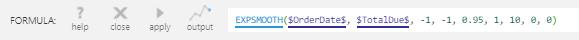Single smoothing formula

Here all the parameters are set to their default values, other than the following three:

• s3 is set to 1 to show the whole results
• s5 is set to 0, which means there is no trend smoothing
• s6 is set to 0, which means there is no season smoothing

The result is a forecasting extension of the Exponential Moving Average.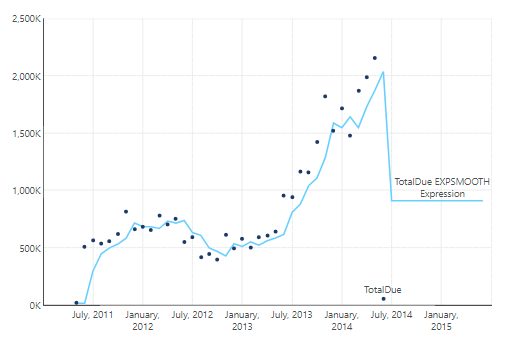Single smoothing formula result

### 5.2. Double exponential smoothing

For some background information on double exponential smoothing, view this article from the National Institute of Standards and Technology.

Open the Data Analysis Panel for the chart and edit the formula for the new measure.

Change the s5 parameter to 1, which will add additive trend smoothing to the single exponential smoothing result. Trend smoothing is an exponential moving average of the slope between points.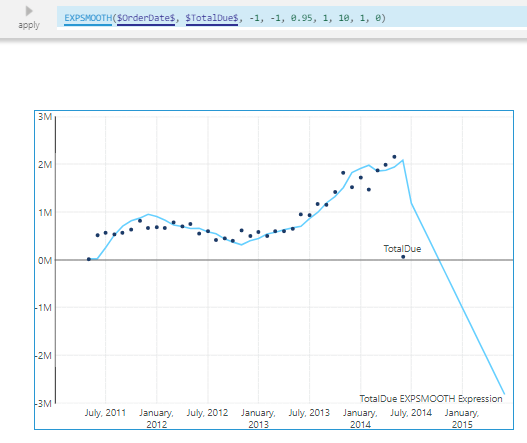Double smoothing formula result

### 5.3. Triple exponential smoothing

For some background information on triple exponential smoothing, view this article from the National Institute of Standards and Technology.

Edit the formula again and change the s6 parameter to 1. This will add additive season smoothing to the double exponential smoothing result. Season smoothing is an exponential moving average of the values over repeating seasons.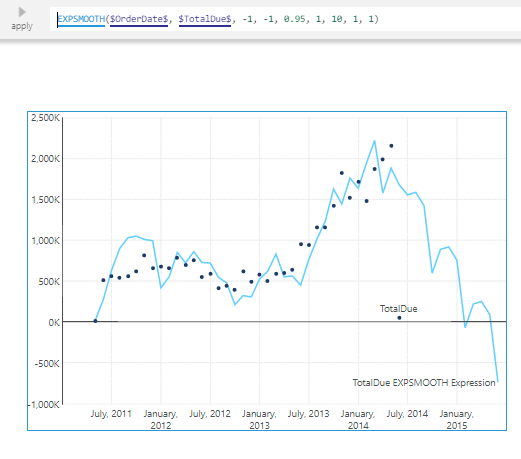Triple smoothing formula result

Notice that the first and last data points are very low and likely throw the forecasting off.

To correct that, switch to view mode, right-click the first data point and select Correct Value. Set the new value to 500000.

Similarly, correct the last data point to 2250000.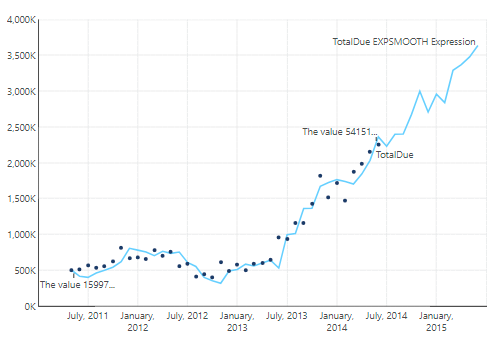Triple smoothing formula result with corrected data

## 6. Notes

• The forecast will only go until the end of the time dimension or until the end of the OLAP time hierarchy.

## 7. See also

Dundas Data Visualization, Inc.
500-250 Ferrand Drive
Toronto, ON, Canada
M3C 3G8

North America: 1.800.463.1492
International: 1.416.467.5100

Dundas Support Hours: 7am-6pm, ET, Mon-Fri